•Sign In
• Hire UsUh Oh! It seems you’re using an Ad blocker!

We always struggled to serve you with the best online calculations, thus, there's a humble request to either disable the AD blocker or go with premium plans to use the AD-Free version for calculators.

Or# Cubic Yard Calculator

Select the area and enter measurements for it. The calculator will estimate the cubic yardage present in it.

Area Shape: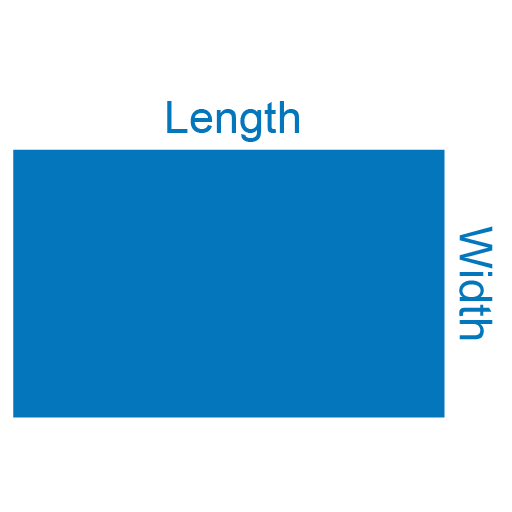Area:

Depth:

Length:

Width:

Flag Thickness:

Side Length a:

Number of steps:

Quantity:

Unit Price (Optional)

\$

Table of Content

 1 What is priceeight Class? 2 priceeight Class Chart: 3 How to Calculate priceeight Density (Step by Step): 4 Factors that Determine priceeight Classification: 5 What is the purpose of priceeight Class? 6 Are mentioned priceeight Classes verified by the officials? 7 Are priceeight Classes of UPS and FedEx same?

Get the Widget!

Add this calculator to your site and lets users to perform easy calculations.

Feedback

How easy was it to use our calculator? Did you face any problem, tell us!

The cubic yard calculator is an online measurement to estimate precisely how much material we need to cover or fill a certain area.
We need to insert the input of length, width, and depth in one unit to estimate the required material for mulch, fill, gravel, cement, or containers. We can calculate the cubic yard volume for landscape material, you can enter the US units like the(inches or feet) or the SI unit like (centimeter or meter) to find the cubic yard. It makes a difference how to figure cubic feet? Or how to figure cubic meters?
The other important thing is the cost of the material which is essential to arranging the budget. We can enter the price or the cost of material per cubic feet, per cubic yards, per cubic meters.

## How to Convert Different Units:

When we are entering a value in the cubic yard calculator whether in the US units or in the metric units. We need to enter the value in some of the following units like inches(in), feet (ft), yards (yd), centimeter (cm), or meter. We need to have complete knowledge of the conversion of some common units to the different units. So we can understand any measurements regardless of the type of the units. It can be difficult how to figure cubic feet from any other units like the cubic meter. We should be familiar with how much is a cubic yard and what its value is in cubic feet

## How to figure cubic feet?

We presenting the various units conversion, and how to figure cubic feet

From feet:

Length (ft) × Width (ft) × Height (ft) = Cubic feet

From inches:

Length (in) × Width (in) × Height (in) ÷ 1728 = Cubic feet

From yards:

Length (yd) × Width (yd) × Height (yd) × 27 = Cubic feet

From cm:

Length (cm) × Width (cm) × Height (cm) ÷ 28316.85 = Cubic feet

From meters:

Length (m) × Width (m) × Height (m) × 35.314667 = Cubic feet

When we are using these above-mentioned formulas, then we are readily able to convert all the units into cubic feet.

## How much is a cubic yard?

To find the cubic yard, we use the formula to reverse the calculation from the cubic feet to cubic yard.

The formula for the cubic yard is as follows:

Cubic yard = Length (ft) x Width (ft) x Depth (ft) ÷ 27

## Why do we need cubic yards?

There should be one question in the mind of the user, why are we measuring the area in the cubic yard, and how much is a cubic yard? The reason behind most of the materials used in the construction is measured in the cubic yard. The common material measured in cubic yards are as follows:

This is the main reason we need to calculate cubic yards regardless of the project. We require a cubic yard calculator to figure out how much material is required for our project. Find out how much you’ll require all the material according to your required length, width and depth. You need to convert and calculate cubic yards.

Remember:

Keep something in your mind:

• 3 feet are in 1 yard exactly
• 27 cubic feet are equal to 1 cubic yard
• 46,656 cubic inches are equal to 1 cubic yard

Cubic feet to Feet Conversion Table

 1 cubic feet = 1 feet 1 feet = 1 cubic feet 2 cubic feet = 1.2599 feet 2 feet = 8 cubic feet 3 cubic feet = 1.4422 feet 3 feet = 27 cubic feet 4 cubic feet = 1.5874 feet 4 feet = 64 cubic feet 5 cubic feet = 1.71 feet 5 feet = 125 cubic feet 6 cubic feet = 1.8171 feet 6 feet = 216 cubic feet 7 cubic feet = 1.9129 feet 7 feet = 343 cubic feet 8 cubic feet = 2 feet 8 feet = 512 cubic feet 9 cubic feet = 2.0801 feet 9 feet = 729 cubic feet 10 cubic feet = 2.1544 feet 10 feet = 1000 cubic feet 11 cubic feet = 2.224 feet 11 feet = 1331 cubic feet 12 cubic feet = 2.2894 feet 12 feet = 1728 cubic feet 13 cubic feet = 2.3513 feet 13 feet = 2197 cubic feet 14 cubic feet = 2.4101 feet 14 feet = 2744 cubic feet 15 cubic feet = 2.4662 feet 15 feet = 3375 cubic feet 16 cubic feet = 2.5198 feet 16 feet = 4096 cubic feet 17 cubic feet = 2.5713 feet 17 feet = 4913 cubic feet 18 cubic feet = 2.6207 feet 18 feet = 5832 cubic feet 19 cubic feet = 2.6684 feet 19 feet = 6859 cubic feet 20 cubic feet = 2.7144 feet 20 feet = 8000 cubic feet 21 cubic feet = 2.7589 feet 21 feet = 9261 cubic feet 22 cubic feet = 2.802 feet 22 feet = 10648 cubic feet 23 cubic feet = 2.8439 feet 23 feet = 12167 cubic feet 24 cubic feet = 2.8845 feet 24 feet = 13824 cubic feet 25 cubic feet = 2.924 feet 25 feet = 15625 cubic feet 26 cubic feet = 2.9625 feet 26 feet = 17576 cubic feet 27 cubic feet = 3 feet 27 feet = 19683 cubic feet 28 cubic feet = 3.0366 feet 28 feet = 21952 cubic feet 29 cubic feet = 3.0723 feet 29 feet = 24389 cubic feet 30 cubic feet = 3.1072 feet 30 feet = 27000 cubic feet

## Cubic yard and area calculation:

We now explain different geometrical shapes and how to calculate cubic yards according to their specific area along with the depth. Keep in mind we first find the area of each geometric shape, then we calculate cubic yards according to each of the geometrical shapes.

## The cubic yard of rectangular area:We first find the area in feet, then find how many yards of volume:

The area of the rectangular area in (feet^2)= Length x Width

The volume (feet^3)= Depth  x Area

The volume in cubic (yard^3)= (volume feet^3)/27

The cubic yard of the rectangular area is measured efficiently by the cubic yards calculator. The most common shape of the yard is usually rectangular and square shapes.

## The cubic yard of square area: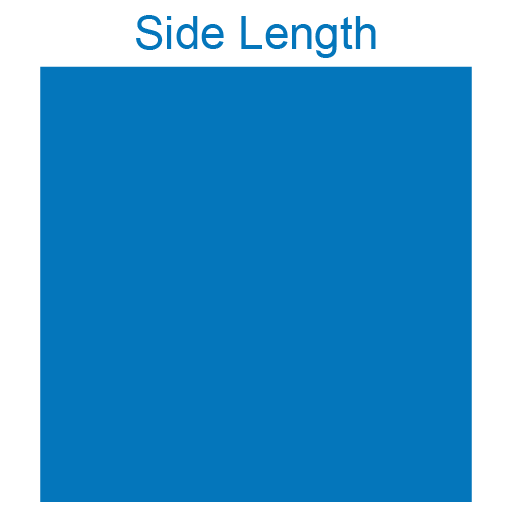We first find the area in feet, then find how many yards of volume:

The area of the square area in (feet^2)= 2 x Side length

The volume (feet^3)= Depth  x Area

The volume in cubic (yard^3)= (volume feet^3)/27

## The cubic yard of rectangular border area: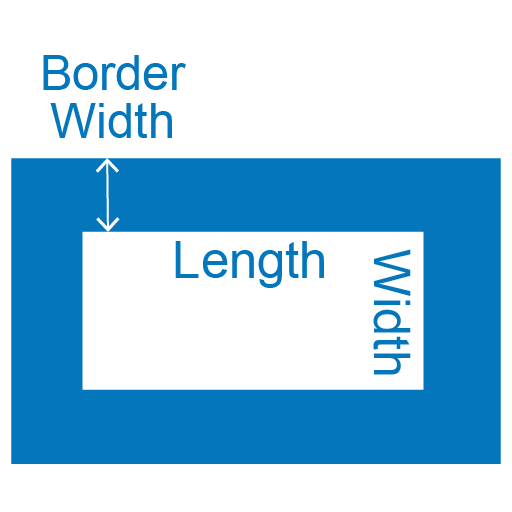We first find the area in feet, then find how many yards of volume:

The area of the inner area (feet^2)= Length x Width

Total area (feet^2) = Length+(2 x Border width)) x(Width +(2 x Border width))

Area (feet^2) = Total area- Inner area

The volume (feet^3)= Depth  x Area

The volume in cubic (yard^3)= (volume feet^3)/27

The cubic yard of the rectangular border area can be measured by the cubic yard calculat<or.

## The cubic yard of a circle: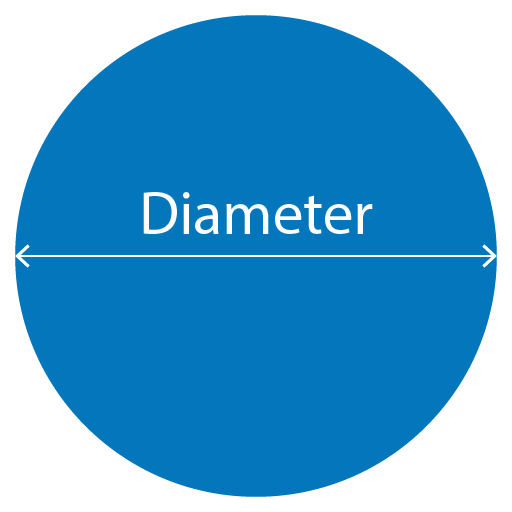The area of the circle area in (feet^2)= Pi x radius^2

Where Pi=3.14

The volume (feet^3)= Depth  x Area

The volume in cubic (yard^3)= (volume feet^3)/27

## The cubic yard of circular border area: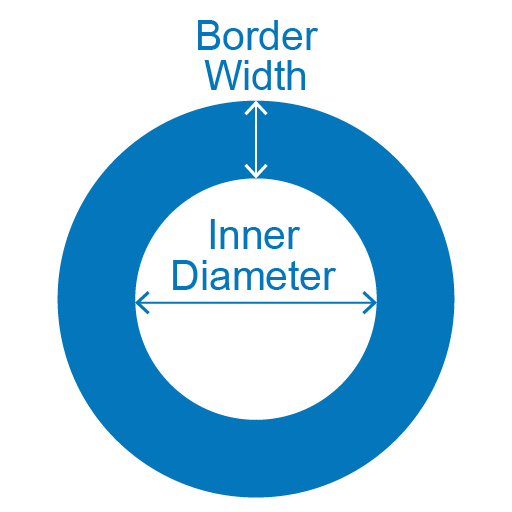The area of the outer diameter(feet^2)= Inner diameter +(2x Border width)

Outer area (feet^2)= Pi x outer radius^2

Inner (feet^2)= Pi x inner radius^2

Where Pi=3.14

Area (feet^2) = outer area- Inner area

The volume (feet^3)= Depth  x Area

The volume in cubic (yard^3)= (volume feet^3)/27

## The cubic yard of circular annulus area: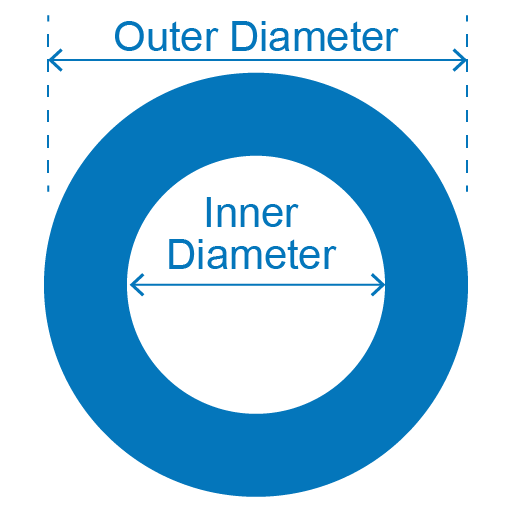The area of the outer diameter(feet^2)= Inner diameter +(2x Border width)

Outer area (feet^2)= Pi x outer radius^2

Inner (feet^2)= Pi x inner radius^2

Where Pi=3.14

Area (feet^2) = outer area- Inner area

The volume (feet^3)= Depth  x Area

The volume in cubic (yard^3)= (volume feet^3)/27

You have observed the yard calculator showing the same result for the circular border area and circular annulus area. Keep in mind we insert different values for calculating cubic yards for both shapes. How many yards of material are required for a circular annulus area can be estimated by the cubic yard calculator.

## The cubic yard of triangular shape: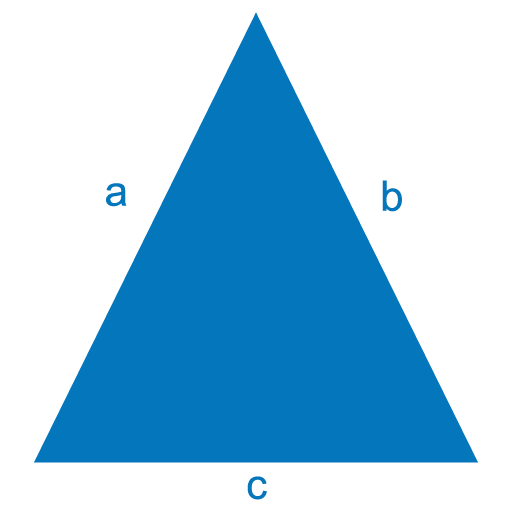The area of the inner area (feet^2)= (¼)x square root[ (a+b+c) x (b+c-a) x (c+a-b) x (a+b-c) ]

The volume (feet^3)= Depth  x Area

The volume in cubic (yard^3)= (volume feet^3)/27

How many yards of material are required for a triangular shape can be measured by the cubic yard calculator.

## The cubic yard of trapezoid shape: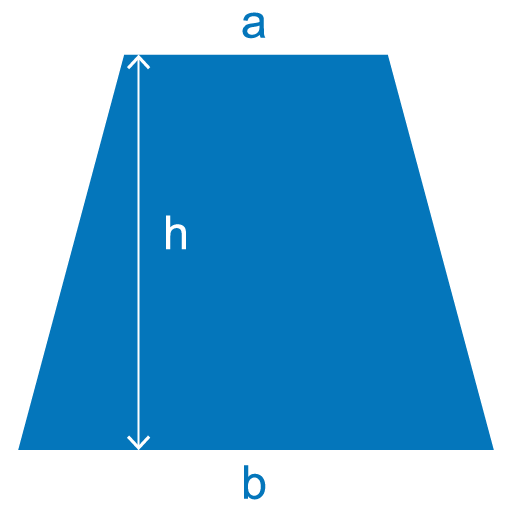The area of the inner area (feet^2)= ((a + b) / 2 )h

The volume (feet^3)= Depth  x Area

The volume in cubic (yard^3)= (volume feet^3)/27

## The cubic yard of the cube: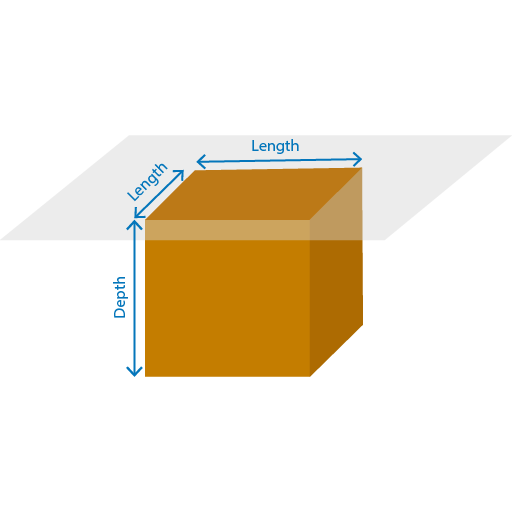The volume (feet^3)=L^3

The volume in cubic (yard^3)= (volume feet^3)/27

Calculating the cubic yards measurement of the cube by the cubic yards calculator is simple and efficient to find the material requirements.

## The cubic yard of  cylinder: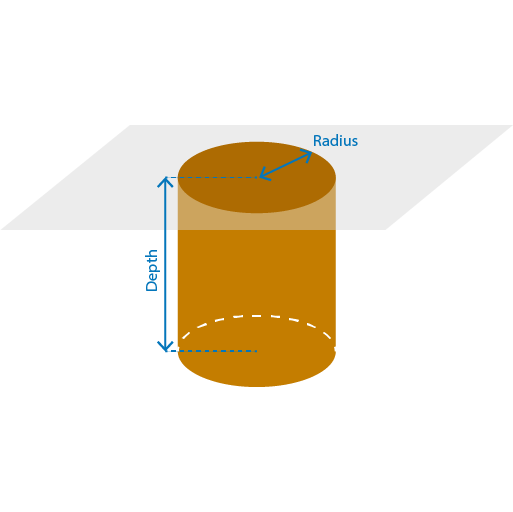The area of the circle area in (feet^2)= Pi x radius^2 x h

Where Pi=3.14

The volume (feet^3)= Depth  x Area

The volume in cubic (yard^3)= (volume feet^3)/27

Calculate the cubic yards capacity of the cylinder by the cubic yard calculator.

## The cubic yard of hollow cylinder: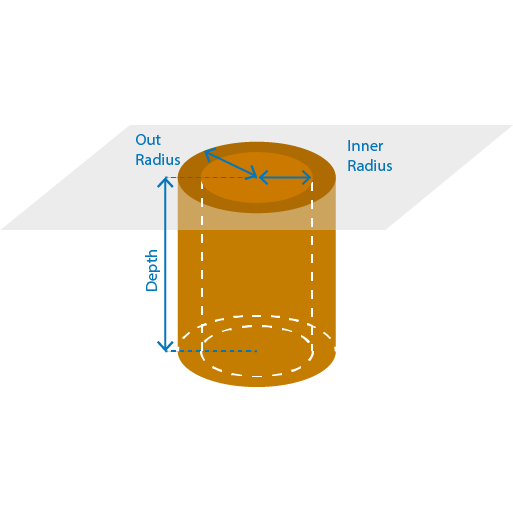The area of the hollow cylinder in (feet^2)=  π (R2 – r2)

Where π =3.14

The volume (feet^3)= Depth  x Area=π (R2 – r2) h

The volume in cubic (yard^3)= (volume feet^3)/27

## The cubic yard of the hemisphere: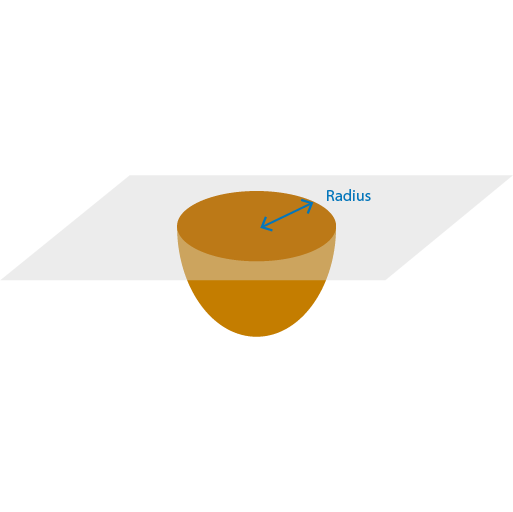The volume (feet^3)= V = (2/3)πr3

The volume in cubic (yard^3)= (volume feet^3)/27

## The cubic yard of the pyramid: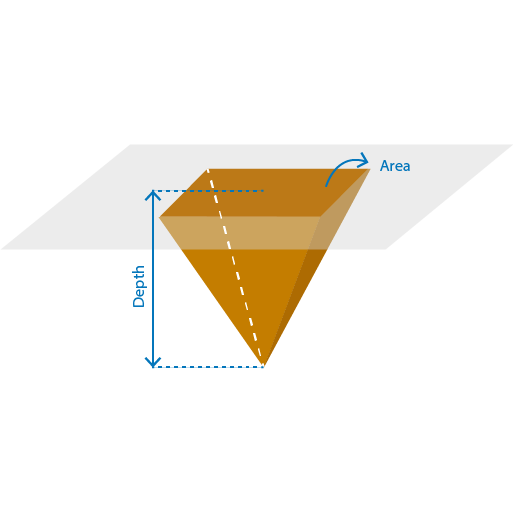he volume (feet^3)= V = (1/3) (Base area x  h)

The base area can be square or rectangular in shape.

The volume in cubic (yard^3)= (volume feet^3)/27

The yard calculator is a simple way to find the cubic yard of a pyramid.

## Working of cubic yard calculator:

The yardage calculator is an efficient way of finding the cubic yard of the most common geometrical shapes. We may need to find out how to figure cubic yards of different geometrical shapes.

Input:

• Select the geometric shape from the drop-down menu
• Enter the required field of your desired geometrical shape
• You can also enter the unit price
• Calculate the cubic yard

Output:

It can be easy to find out the construction material with the help of the cubic yard calculator.

• The result is represented in cubic yard
• There can be other units also displayed

## FAQs:

### How do you find square yards of dirt?

For adding 1 foot of soil to the area, take the total square area, multiply it by one, then divide by 27 to convert the square feet into square yards.

### How many yards of concrete do I require for a 24×24 slab?

We need around 7.11 yards. This can be determined by the cubic yard calculator

## Conclusion:

When we are using the cubic yard calculator, we can estimate the required concrete and the other material required for the whole construction site. It is essential for operation management and estimation of the total cost of our project.

## References:

From the source of Wikipedia: Cubic yard, Cubic yard per second, Cubic yard per minute

From the source of Wikihow: How to determine the cubic yards, Obtain necessary measurements